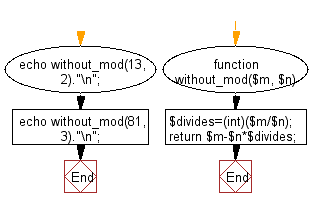﻿ PHP Exercise: Calculate the mod of two given integers without using any inbuilt modulus operator - w3resource# PHP Exercises: Calculate the mod of two given integers without using any inbuilt modulus operator

## PHP: Exercise-40 with Solution

Write a PHP program to calculate the mod of two given integers without using any inbuilt modulus operator.

Sample Solution: -

PHP Code:

``````<?php
function without_mod(\$m, \$n)
{
\$divides=(int)(\$m/\$n);
return \$m-\$n*\$divides;
}
echo without_mod(13, 2)."\n";
echo without_mod(81, 3)."\n";
?>
```
```

Sample Output:

```1
0
```

Flowchart:PHP Code Editor:

Have another way to solve this solution? Contribute your code (and comments) through Disqus.

What is the difficulty level of this exercise?

﻿

## PHP: Tips of the Day

PHP: PHP Constants Containing Arrays?

NOTE: while this is the accepted answer, it's worth noting that in PHP 5.6+ you can have const arrays

You can also serialize your array and then put it into the constant:

```# define constant, serialize array
define ("FRUITS", serialize (array ("apple", "cherry", "banana")));

# use it
\$my_fruits = unserialize (FRUITS);
```

Ref : https://bit.ly/2EcIer8